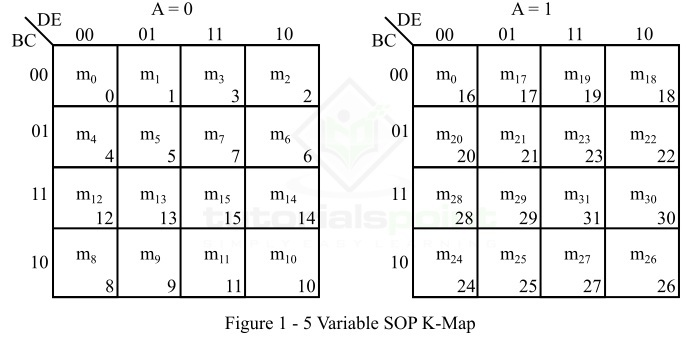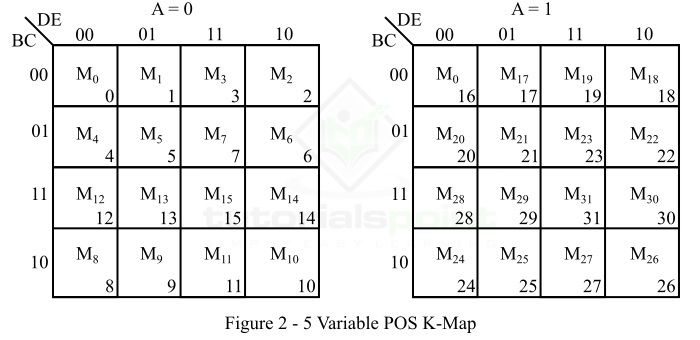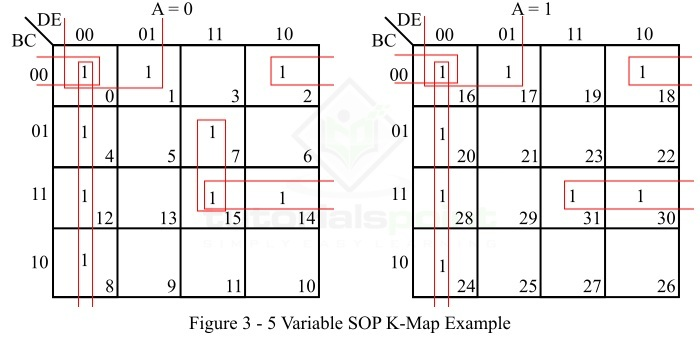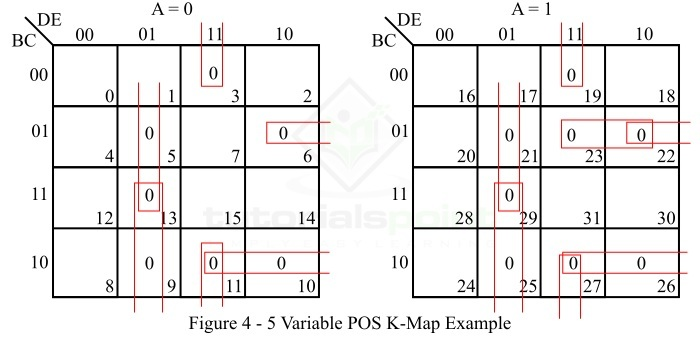# 5 Variable K-Map in Digital Electronics

K-Map or Karnaugh Map is a simplification technique used to minimize a given complex Boolean function. K-Map or Karnaugh Map is a graph or chart which is composed of an arrangement of adjacent cells, where each cell of the K-Map represents a particular combination of variables in either sum or product form. The K-map can be used to simplify Boolean functions involving any number of variables. But, the simplification of a Boolean function using K-map becomes very complex for expressions involving five or more variables. Therefore, in actual practice, the K-map is limited to six variables.

The number of cells in a K-map depends upon the number of variables in the given Boolean function. A K-map will have 2n cells or squares, where n is the number of variables in the Boolean expression. Therefore, for a two variable function, the K-map will have 22 = 4 cells, for a three variable Boolean function, the K-map will have 23 = 8 cells, and for four variable Boolean function, the K-map will have 24 = 16 cells, and so on.

In this tutorial, we will discuss five variable K-Map and will use it to simplify Boolean functions in 5 variables. So let’s start with the introduction of 5 variable K-map.

## Five Variable K-Map

A five variable K-map is used to minimize a 5-variable Boolean expression to its reduced form. The following are the important characteristics of a 5 variable K-map −

• A five variable K-map have 32 (25) cells or squares, and each cell of the K-map represents either a minterm or a maxterm of the Boolean expression.

• If the given Boolean function is expressed in SOP (Sum of Products) form, then the minterms of five variables Boolean function are designated as m0,m1,m2,m3…m31. Where, m0 is corresponding to $\mathrm{\lgroup\overline{A}\:\overline{B}\:\overline{C}\:\overline{D}\:\overline{E} \rgroup}$, m1 is corresponding to $\mathrm{\lgroup\overline{A}\:\overline{B}\:\overline{C}\:\overline{D}E\rgroup}$,… and m31 is corresponding to $\mathrm{\lgroup ABCDE\rgroup}$.

• On the other hand, if the 5 variable Boolean function is expressed in POS (Product of Sums) form, then the maxterms of the function are designated as M0, M1, M2,… M31. Where, M0 represents $\mathrm{\lgroup A+B+C+D+E\rgroup}$, M1 represents $\mathrm{\lgroup A+B+C+D+\overline{E}\rgroup}$,… and M31 represents $\mathrm{\lgroup\overline{A}+\overline{B}+\overline{C}+\overline{D}+\overline{E} \rgroup}$.

The 32 cells of the five variable K-map are divided into two blocks of 16 cells each, which are arranged side by side. The left block represents minterms (or maxterms) from m0 to m15 (or M0 to M15. Wheck, thck, th). In the left block, the first variable (let A) is a 0. The right block represents minterms (or maxterms) from m16 to m31 (or M16 to M31), in this block A is a 1.

In the five variable K-map, we can form 2-squares, 4-squares, 8-squares, 16-squares, or 32-squares by involving its two blocks. Also, squares are considered adjacent in these two blocks, when one block superimposes on the top of another.

A five variable SOP K-map is shown in Figure 1.A five variable POS K-map is represented in Figure 2.Now, let us discuss some solved examples to understand the application of a 5 variable K map in reducing a given 5-variable Boolean function in either SOP form or POS form.

## Example 1

Reduce the following 5 variable Boolean function in SOP form using the five variable K map.

$$\mathit{f}\mathrm{\lgroup A,B,C,D,E\rgroup=\sum m\lgroup 0,1,2,4,7,8,12,14,15,16,17,18,20,24,28,30,31\rgroup }$$

### Solution

The SOP K-map representation of the given SOP Boolean function is shown in Figure 3.### Explanation

The minimization of the given 5 variable Boolean function using the five variable K-map (Figure 3) is done as per the following steps −

• There are no isolated 1s in the K-map.

• The minterm m0 can form an 8-square with m4, m8, m12, m16, m20, m24, and m28. So make it and read it as $\mathrm{\lgroup\overline{D}\:\overline{E} \rgroup}$.

• The minterms m0, m1, m16, and m17 form a 4-square. Make it and read it as $\mathrm{\lgroup\overline{B}\:\overline{C}\:\overline{D} \rgroup}$.

• The minterms m0, m2, m16, and m18 form a 4-square. Make it and read it as $\mathrm{\lgroup\overline{B}\:\overline{C}\:\overline{E} \rgroup}$.

• The minterms m7 and m15 form a 2-square. Make it and read it as $\mathrm{\lgroup\overline{A}CDE \rgroup}$.

• The minterms m14, m15, m30 and m31 form a 4-square. Make it and read it as $\mathrm{\lgroup BCD \rgroup}$.

• Finally, write all the product terms in SOP form.

Hence, the minimal SOP expression of the given 5 variable Boolean function is,

$$\mathit{f}\mathrm{(A,B,C,D,E)=\overline{A}CDE+\overline{B}\:\overline{C}\:\overline{D}+\overline{B}\:\overline{C}\:\overline{E}+BCD+\:\overline{D}\:\overline{E}}$$

## Example 2

Minimize the following 5 variable Boolean function in POS form using the five variable K map.

$$\mathit{f}\mathrm{\lgroup A,B,C,D,E\rgroup=\prod M\lgroup 3,5,6,9,10,11,13,19,21,22,23,25,26,27,29\rgroup}$$

### Solution

The POS K-map representation of the given POS Boolean function is shown in Figure 4.### Explanation

The minimization of the given 5 variable Boolean function using the five variable K-map (figure-4) is done as per the following steps −

• There are no isolated zeros in the K-map.

• The maxterms M9, M13, M25, and M29 form a 4 – square. Make it and read it as $\mathrm{\lgroup\overline{B}+D+\overline{E} \rgroup}$.

• The maxterms M3, M11, M19, and M27 form a 4 – square. Make it and read it as $\mathrm{\lgroup C+\overline{D}+\overline{E} \rgroup}$.

• The maxterms M5, M13, M21, and M29 form a 4 – square. Make it and read it as $\mathrm{\lgroup \overline{C}+D+\overline{E} \rgroup}$.

• The maxterms M6 and M22 form a 2 – square. Make it and read it as $\mathrm{\lgroup B+\overline{C}+\overline{D}+E \rgroup}$.

• The maxterms M10, M11, M26, and M27 form 4 – square. Make it and read it as $\mathrm{\lgroup \overline{B}+C+\overline{D} \rgroup}$.

• The maxterms M22 and M23 form 2 – square. Make it and read it as $\mathrm{\lgroup \overline{A}+B+\overline{C}+\overline{D} \rgroup}$.

• Finally, write all the sum terms in POS form.

Therefore, the minimal POS expression of the given Boolean function in five variables is,

$$\mathit{f}\mathrm{\lgroup A,B,C,D,E\rgroup=\lgroup \overline{B}+D+\overline{E}\rgroup \lgroup C+\overline{D}+\overline{E}\rgroup\lgroup \overline{C}+D+\overline{E}\rgroup\lgroup B+\overline{C}+\overline{D}+E\rgroup\lgroup \overline{B}+C+\overline{D}\rgroup\lgroup \overline{A}+B+\overline{C}+\overline{D}\rgroup}$$

## Tutorial Problems

Try to solve the following tutorial problems to get better command on the utilization of five variable K-map to reduce a Boolean expression.

Q1.Reduce the following five variable Boolean expression in SOP form using K-Map.

$$\mathit{f}\mathrm{\lgroup A,B,C,D,E\rgroup=\sum m\lgroup 0,3,4,6,8,10,11,12,15,17,18,22,25,26,27,30,31\rgroup }$$

Q2.Reduce the following five variable Boolean expression in POS form using K-map.

$$\mathit{f}\mathrm{\lgroup A,B,C,D,E\rgroup=\prod M\lgroup 0,1,2,4,6,7,9,10,11,13,15,16,18,19,25,26,28,29,31\rgroup }$$

## Conclusion

This is all about the five variable K-map. From the above discussion, we can conclude that a five variable Boolean function can be reduced to the minimal form using the 5 variable K-map. A five variable K-map has 32 squares or cells from 0 to 31. These 32 cells are arranged in two blocks 16 cells each. However, the split form of the 5 variable K-map into two blocks makes the use of it to minimize a Boolean function slightly complex.

Updated on: 03-Oct-2023

733 Views